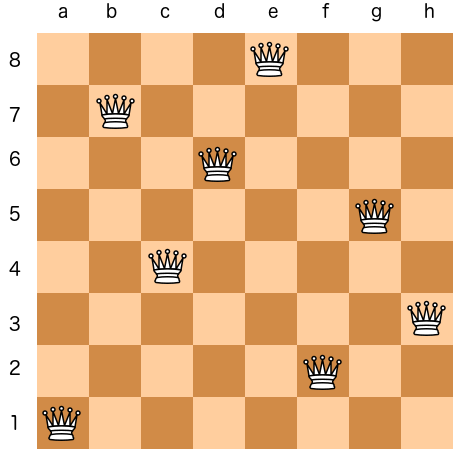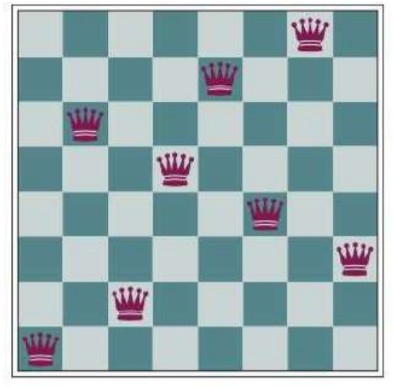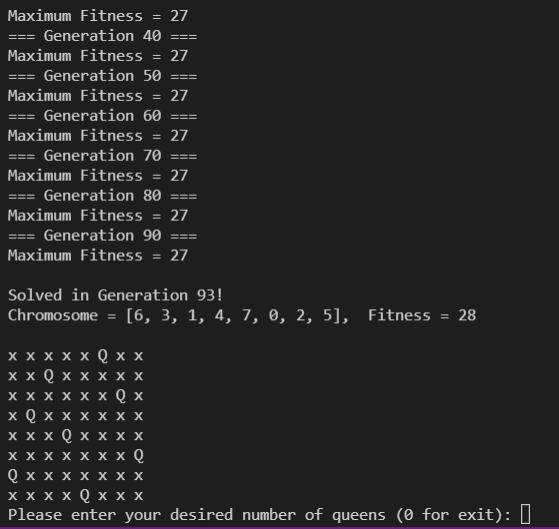# N-Queen-Problem-using-Genetic-Algorithm

The N Queen is the problem of placing N chess queens on an N×N chessboard so that no two queens attack each other. For example, following is a solution for 8 Queen problem.The 8 queens problem is simple. On an 8×8 chess board, the queen can move any number of squares horizontally, vertically, and diagonally. Normally, there is one queen per side on the board but for this problem, there are 8. This can be generalized to N queens on an NxN board. The goal is to place 8 queens on the board such that every pair of queens aren’t attacking each other. In the picture above, you can see that every queen is not in the line of sight of another queen. Here’s an example of a board state that is not the solution:The pair of queens on the 4th and 7th columns are attacking each other diagonally.

An example of exacution:View Github